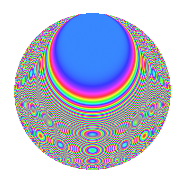# Properties

 Label 756.2.oLevel 756 Weight 2 Character orbit o Rep. character $$\chi_{756}(179,\cdot)$$ Character field $$\Q(\zeta_{6})$$ Dimension 88 Newform subspaces 1 Sturm bound 288 Trace bound 0

# Related objects

## Defining parameters

 Level: $$N$$ = $$756 = 2^{2} \cdot 3^{3} \cdot 7$$ Weight: $$k$$ = $$2$$ Character orbit: $$[\chi]$$ = 756.o (of order $$6$$ and degree $$2$$) Character conductor: $$\operatorname{cond}(\chi)$$ = $$252$$ Character field: $$\Q(\zeta_{6})$$ Newform subspaces: $$1$$ Sturm bound: $$288$$ Trace bound: $$0$$

## Dimensions

The following table gives the dimensions of various subspaces of $$M_{2}(756, [\chi])$$.

Total New Old
Modular forms 312 104 208
Cusp forms 264 88 176
Eisenstein series 48 16 32

## Trace form

 $$88q + 3q^{2} + q^{4} + O(q^{10})$$ $$88q + 3q^{2} + q^{4} + 2q^{10} - 4q^{13} + 3q^{14} + q^{16} + 6q^{20} - 6q^{22} - 60q^{25} + 6q^{26} + 24q^{29} - 27q^{32} - 4q^{34} - 4q^{37} + 8q^{40} + 12q^{41} + 57q^{44} - 6q^{46} - 2q^{49} - 9q^{50} + 14q^{52} + 66q^{56} - 10q^{58} + 2q^{61} - 8q^{64} - 18q^{65} + 30q^{70} - 4q^{73} - 6q^{76} + 30q^{77} - 87q^{80} - 4q^{82} - 14q^{85} - 18q^{88} - 60q^{89} - 24q^{92} + 9q^{94} - 4q^{97} + 57q^{98} + O(q^{100})$$

## Decomposition of $$S_{2}^{\mathrm{new}}(756, [\chi])$$ into newform subspaces

Label Dim. $$A$$ Field CM Traces $q$-expansion
$$a_2$$ $$a_3$$ $$a_5$$ $$a_7$$
756.2.o.a $$88$$ $$6.037$$ None $$3$$ $$0$$ $$0$$ $$0$$

## Decomposition of $$S_{2}^{\mathrm{old}}(756, [\chi])$$ into lower level spaces

$$S_{2}^{\mathrm{old}}(756, [\chi]) \cong$$ $$S_{2}^{\mathrm{new}}(252, [\chi])$$$$^{\oplus 2}$$

## Hecke Characteristic Polynomials

There are no characteristic polynomials of Hecke operators in the database